Question

A wave passes your house which has originated at a distance of x = 15m away from your house at the time t = 0s. It is traveling at a speed of 50 m/s. The displacement of the wave as it passes your house is 4m. It has a frequency of 2Hz. You also notice that the maximum displacement of the wave is 5m.

(a) Write the equation for the displacement of this wave as a function of position and time.

(b) Draw a history graph (at x = 0m) and a snapshot graph (at t = 0s).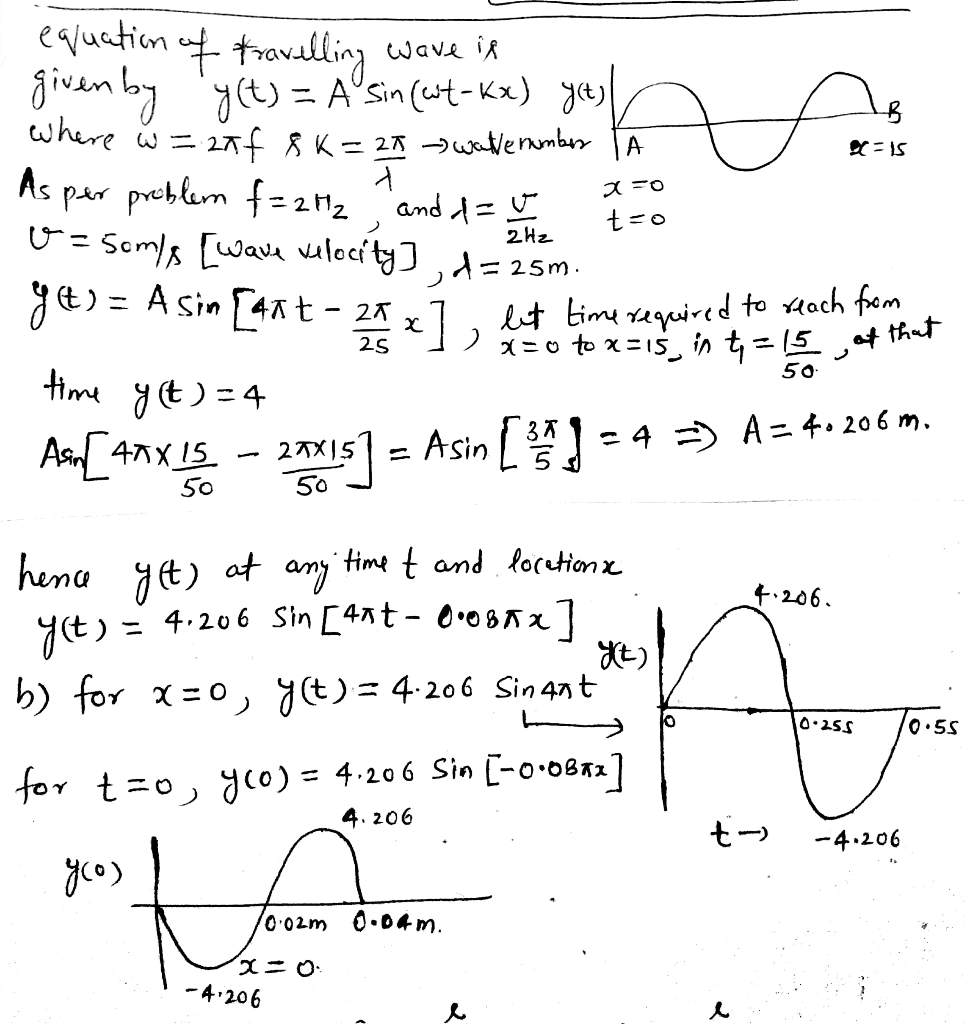#### Earn Coins

Coins can be redeemed for fabulous gifts.

Similar Homework Help Questions
• ### A wave passes your house (considered to be x = 0), and you start your stopwatch...

A wave passes your house (considered to be x = 0), and you start your stopwatch as the wave passes (marking t = 0). It is traveling at a speed of 50 m/s in the negative-x direction. The displacement of the wave as it passes your house is 4 m even though its amplitude is 6m. It has a frequency of 2 Hz. (a) Write the equation for the displacement of this wave as a function of position and time....

• ### (2) A wave passes your house (considered to be x “ 0), and you start your...

(2) A wave passes your house (considered to be x “ 0), and you start your stopwatch as the wave passes (marking t “ 0). It is traveling at a speed of 50 m/s in the negative-x direction. The displacement of the wave as it passes your house is 4 m even though its amplitude is 6m. It has a frequency of 2 Hz. (a) Write the equation for the displacement of this wave as a function of position and...

• ### (1) A wave is traveling in the positive-y direction with wavelength λ “ 12 m and...

(1) A wave is traveling in the positive-y direction with wavelength λ “ 12 m and frequency f “ 1 6 Hz. At t “ 0, there is no displacement of the medium at the origin, but its amplitude is 6m. (a) Write the equation for the displacement of this wave as a function of position and time. (b) Draw a quantitatively accurate history graph (at y “ 3m) and snapshot graph (at t “ 2s). (c) What is the...

• ### (1) A wave is traveling in the positive y direction with wavelength λ = 12 m...

(1) A wave is traveling in the positive y direction with wavelength λ = 12 m and frequency f = 1/6 Hz. At t = 0, there is no displacement of the medium at the origin, but its amplitude is 6m. (a) Write the equation for the displacement of this wave as a function of position and time. (b) Draw a quantitatively accurate history graph (at y = 3m) and snapshot graph (at t = 2s). (c) What is the...

• ### 1. The left figure is a history graph that shows the displacement D of a traveling wave at a given position as a function of time. The right figure is a snapshot graph that shows the displacement...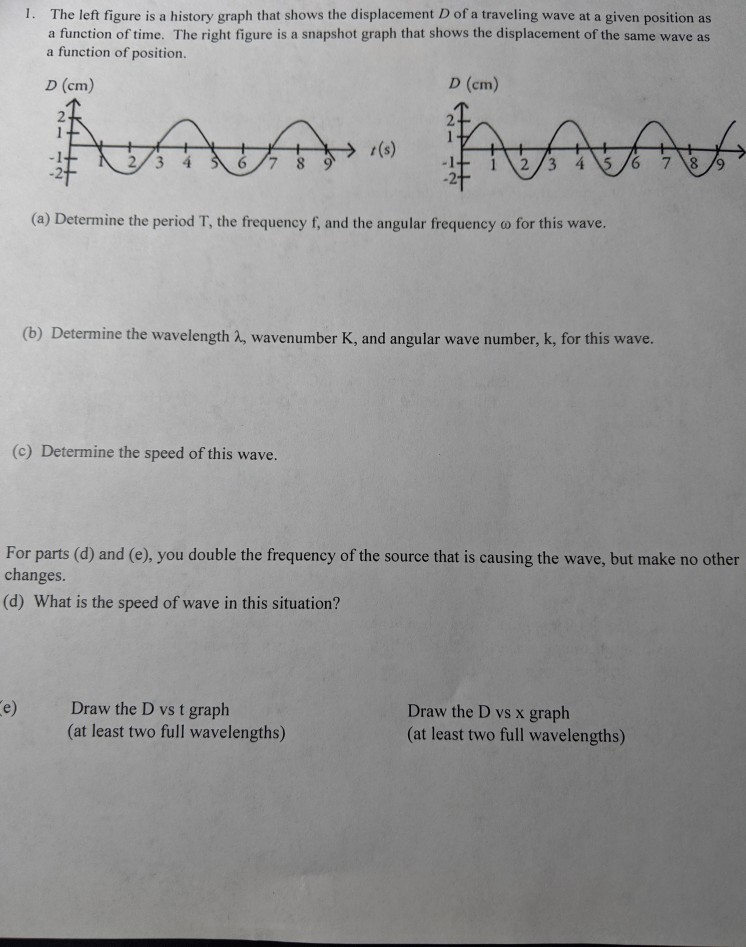1. The left figure is a history graph that shows the displacement D of a traveling wave at a given position as a function of time. The right figure is a snapshot graph that shows the displacement of the same wave as a function of position. D (cm) D (cm) -1 -2 2/3 4 67 8 -2 (a) Determine the period T, the frequency f, and the angular frequency o for this wave. (b) Determine the wavelength 2, wavenumber K,...

• ### (1) A wave is traveling in the positive-y direction with wavelength λ f Hz. At t...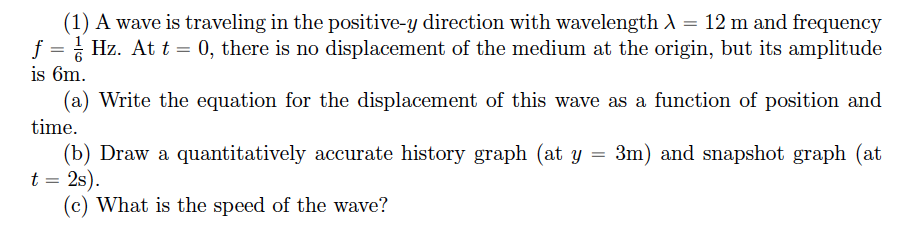(1) A wave is traveling in the positive-y direction with wavelength λ f Hz. At t is 6m 12 m and frequency 0, there is no displacement of the medium at the origin, but its amplitude a) Write the equation for the displacement of this wave as a function of position and (b) Draw a quantitatively accurate history graph (at y 3m) and snapshot graph (at t 2s) (c) What is the speed of the wave?

• ### I provided a close up of the graph. Any help on this question would be great....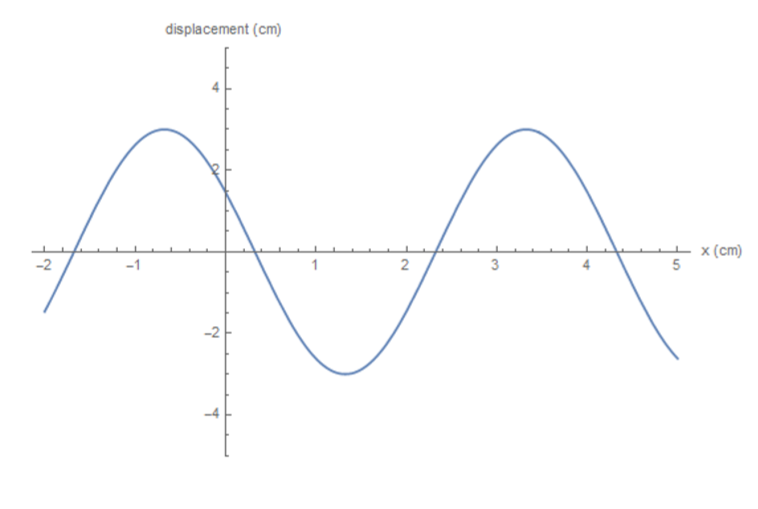I provided a close up of the graph. Any help on this question would be great. displacement (cm) x(cm) -2 -1 2 -2 -4 From the following snapshot taken at a time of t(1/2) T of a wave traveling to the right with a velocity of 24 cm/s. a. Find the Amplitude, wavelength, frequency, and phase constant. (use sine function) b. Write the numerical equation for the displacement of this wave. c. Write the equation for the velocity and acceleration...

• ### Wave function You are observing a wave traveling along the x-axis. The first picture (y vs....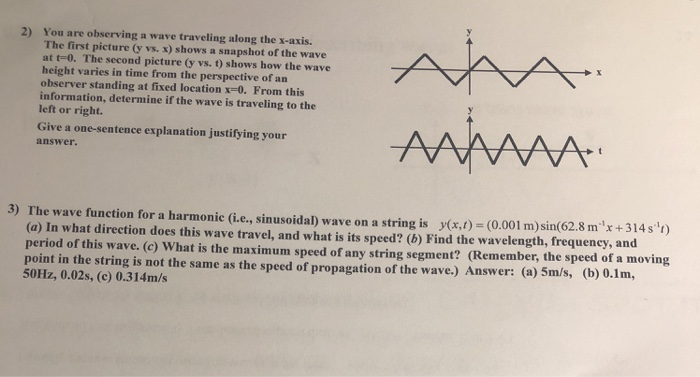Wave function You are observing a wave traveling along the x-axis. The first picture (y vs. x) shows a snapshot of the wave at t=0. The second picture dy vs. t) shows how the wave height varies in time from the perspective of an observer standing at fixed location x-0. From this information, determine if the wave is traveling to the left or right. Give a one-sentence explanation justifying your answer 2) 3) The wave function for a harmonic (i.e.,...

• ### The graphs below show a snapshot of a wave on a string at time t =...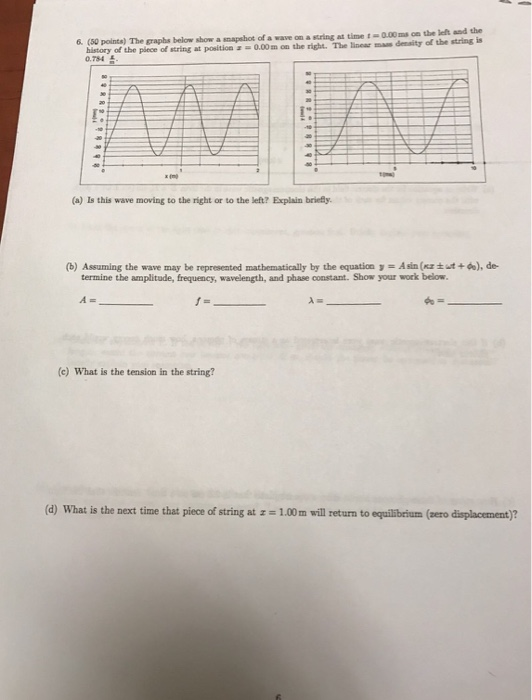The graphs below show a snapshot of a wave on a string at time t = 0.00 ms on the left and the history of the piece of string at position x = 0.00 m on the right. The linear mass density of the string is 0.784 t/m. Is this wave moving to the right or to the left? Explain briefly. Assuming the wave may be represented mathematically by the equation y = A sin (kx plusminus omega t +...

• ### The snapshot graph at t=1 s and the history graph at x=1 cm of a wave...The snapshot graph at t=1 s and the history graph at x=1 cm of a wave are shown below (snapshot on left, history on right). What are the amplitude, frequency, and speed of the wave? Amplitude =    cm Frequency =    Hz    Speed = cm/s What is the equation of this wave, expressed as y=f(x,y)? Express the coefficients of the x and t terms as decimals (i.e. don’t use π in your equation, use 3.14159...), and ignore units....